Contemporary Mathematics

# Chapter 5

5.1
1.
18 plus 11 OR the sum of 18 and 11
2.
27 times 9 OR the product of 27 and 9
3.
84 divided by 7 OR the quotient of 84 and 7
4.
$p$ minus $q$ OR the difference of $p$ and $q$
5.2
1.
$43 + 67$
2.
$\left( {45} \right)\left( 3 \right)$
3.
$45 \div 3$
4.
$89-42$
5.3
1.
$n - 20$
2.
$\left( {n + 2} \right) \bullet 6$ OR $6\left( {n + 2} \right)$
3.
${n^3} - 5$
4.
$60h + 40$
5.4
1.
$5y = 50$
2.
$\frac{1}{2}n = 30$
3.
$3n - 7 = 2$
4.
$2x + 7 = 21$
5.5
1.
$24 - \left( {17 - 6} \right) = 13$
2.
$\left( {3 \bullet 6} \right) + 13 = 31$
3.
$\left( {12 - 6} \right) \div \left( {5 - 3} \right) = 3$
4.
$5 \bullet \left( {{3^2} + 5} \right) = 70$
5.6
1.
$9$
2.
$- 6$
5.7
1.
$5{x^{ 2}}-3x - 7$
5.8
1.
$- 9x + 4$
5.9
1.
$2y + 10$
2.
$- 2a - 2b + 8$
3.
$112 + 16x$
4.
$6x + 18$
5.
$3a + 4$
5.10
1.

$2{x^2} - 11x + 12$

5.11
1.

$4{x^2} + x - 2$

5.12
1.
$x = 3$
5.13
1.
$y = 28$
5.14
1.
Henry has 42 books.
5.15
1.
Total cost is $48.85 5.16 1. Answers will vary. For example: You can rent a paddleboard for$25 per hour with a water shoe purchase of $75. If you spent$200, how many hours did you rent the paddle board for?
You rented the paddle board for 5 hours.
5.17
1.
$12 = - 1$, which is false; therefore, this is a false statement, and the equation has no solution.
5.18
1.
$- 12 = - 12$, which is true; therefore, this is a true statement, and there are infinitely many solutions.
5.19
1.
$t = \frac{I}{{\text{P}}r}$
5.20
1.
$h = \frac{{3V}}{{\pi {r^2}}}$
5.21
1.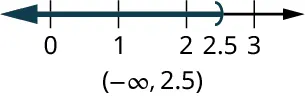5.22
1.
$0 \leq x \leq 2.5$
Graph: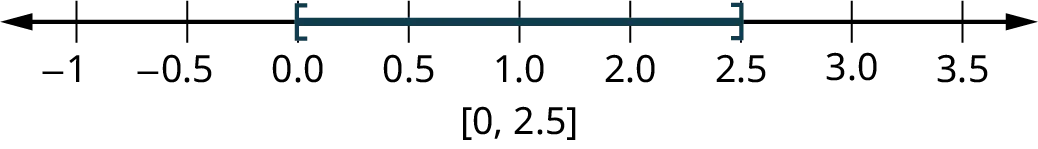5.23
1.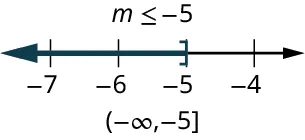5.24
1.

$p \geq 2$5.25
1.
Taleisha can send/receive 106 or fewer text messages and keep her monthly bill no more than $50. 5.26 1. You could take up to 17 credit hours and stay under$2,000.
5.27
1.
Malik must tutor at least 23 hours.
5.28
1.
$a$ = 1 U.S. dollar, and $b$ = 1.21 Canadian dollars, the ratio is 1 to 1.21; or 1:1.21; or $\frac{1}{{1.21}}$ .
5.29
1.
With $a$ = 170 pounds on Earth, and $b$ = 64 pounds on Mars, the ratio is 170 to 64; or 170:64; or $\frac{{170}}{{64}}$.
5.30
1.
$219.51 5.31 1. 501.6 pounds 5.32 1. 720 cookies 5.33 1. The constant of proportionality (centimeters divided by inches) is 2.54. This tells you that there are 2.54 centimeters in one inch. 5.34 1. 30.5 hours (or 30½ hours, or 30 hours and 30 minutes) 5.35 1.$207.50
5.36
1.
125.9 miles
5.37
1.
The scale is $1{\text{ inch}} = 91.25{\text{ miles}}$. The other borders would calculate as: eastern and western borders are 273.75 miles, and northern border is 365 miles.
5.38
1.
184 inches, or 15 feet, 4 inches.
5.39
1.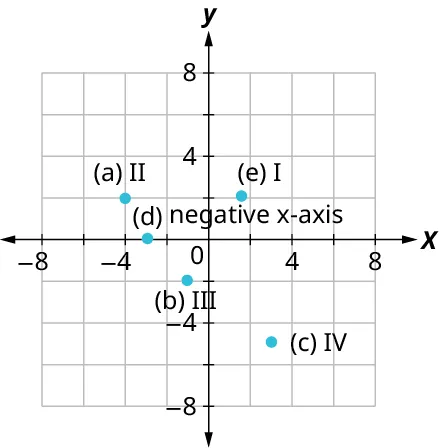5.40
1.

1. Yes
2. Yes
2.

1. No
2. No
3.

1. Yes
2. Yes
4.

1. Yes
2. Yes
5.41
1.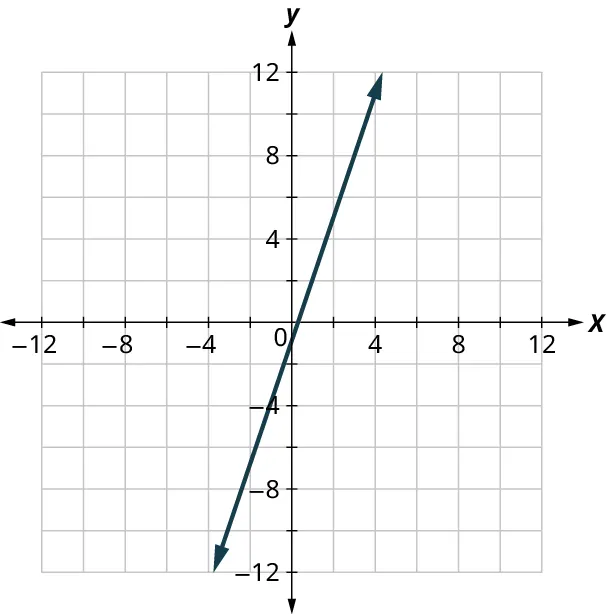5.42
1.Your friend will pay $1.65. 5.43 1. Yes 2. Yes 3. No 4. No 5. No 5.44 1. $y \geq - 2x + 3$ 5.45 1.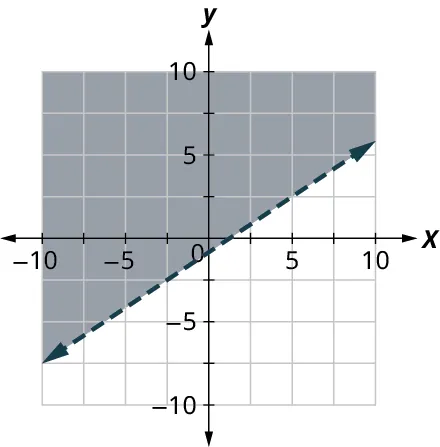5.46 1. $11x + 16.5y \geq 330$ 2.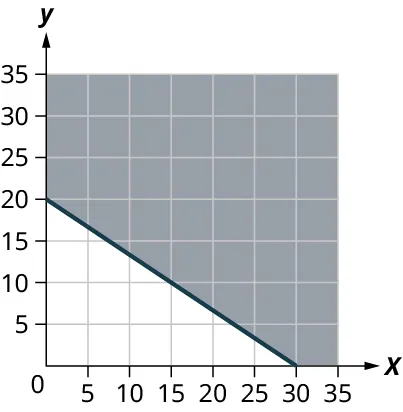3. Answers will vary. 5.47 1. ${x^2} + 4x + 3$ 5.48 1. $2{x^2} - 5x - 3$ 5.49 1. $\left( x + 2 \right)\left( x + 4 \right)$ 5.50 1. $\left( {x - 7} \right)\left( {x - 9} \right)$ 5.51 1.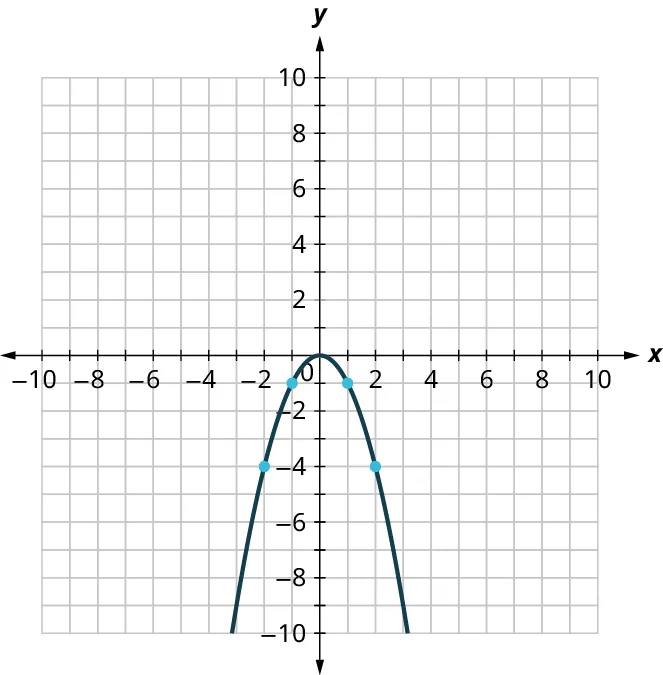5.52 1. $x = - 3,\,\,x = 2$ 5.53 1. $x = 2$ or $x = - 5$ 5.54 1. $x = 3$ or $x = - 5$ 5.55 1. $x = 5$, $x = -5$ 5.56 1. $p = \frac{7}{5}$, $p = - \frac{7}{5}$ 5.57 1. $a = 5$, $a = - 3$ 5.58 1. $y = 1$, $y = \frac{2}{3}$ 5.59 1. 16, 15 and –16, –15 5.60 1. ${\text{width}} = 5{\text{ feet}}$, ${\text{length}} = 6{\text{ feet}}$ 5.61 1. 22 2. 6 3. $3{t^2} - 2t + 1$ 5.62 1. There 205 unread emails after 7 days. 5.63 1. This relation is a function. 2. This relation is not a function. 5.64 1. Both George and Mike have two phone numbers. Each $x$-value is not matched with only one $y$-value. This relation is not a function. 5.65 1. function 2. not a function 3. function 5.66 1. This graph represents a function. 5.67 1. This graph does not represent a function. 5.68 1. The domain is the set of all $x$-values of the relation: $\left\{ {1,2,3,4,5} \right\}$. 2. The range is the set of all $y$-values of the relation: $\left\{ {1,8,27,64,125} \right\}$. 5.69 1. The ordered pairs of the relation are: $\left\{ {\left( {-3,\,3} \right),\left( {-2,\,2} \right),\left( {-1,\,0} \right),\left( {0,\,-1} \right),\left( {2,\,-2} \right),\left( {4,\,-4} \right)} \right\}$. 2. The domain is the set of all $x$-values of the relation: $\left\{ {-3,-2,-1,0,2,4} \right\}$. 3. The range is the set of all $y$-values of the relation: $\left\{{-4,-2,-1,0,2,3}\right\}$. 5.70 1. The graph crosses the $x$-axis at the point (2, 0). The $x$-intercept is (2, 0). The graph crosses the $y$-axis at the point (0, −2). The $y$-intercept is (0, −2). 5.71 1. The $x$-intercept is (4, 0) and the $y$-intercept is (0, 12).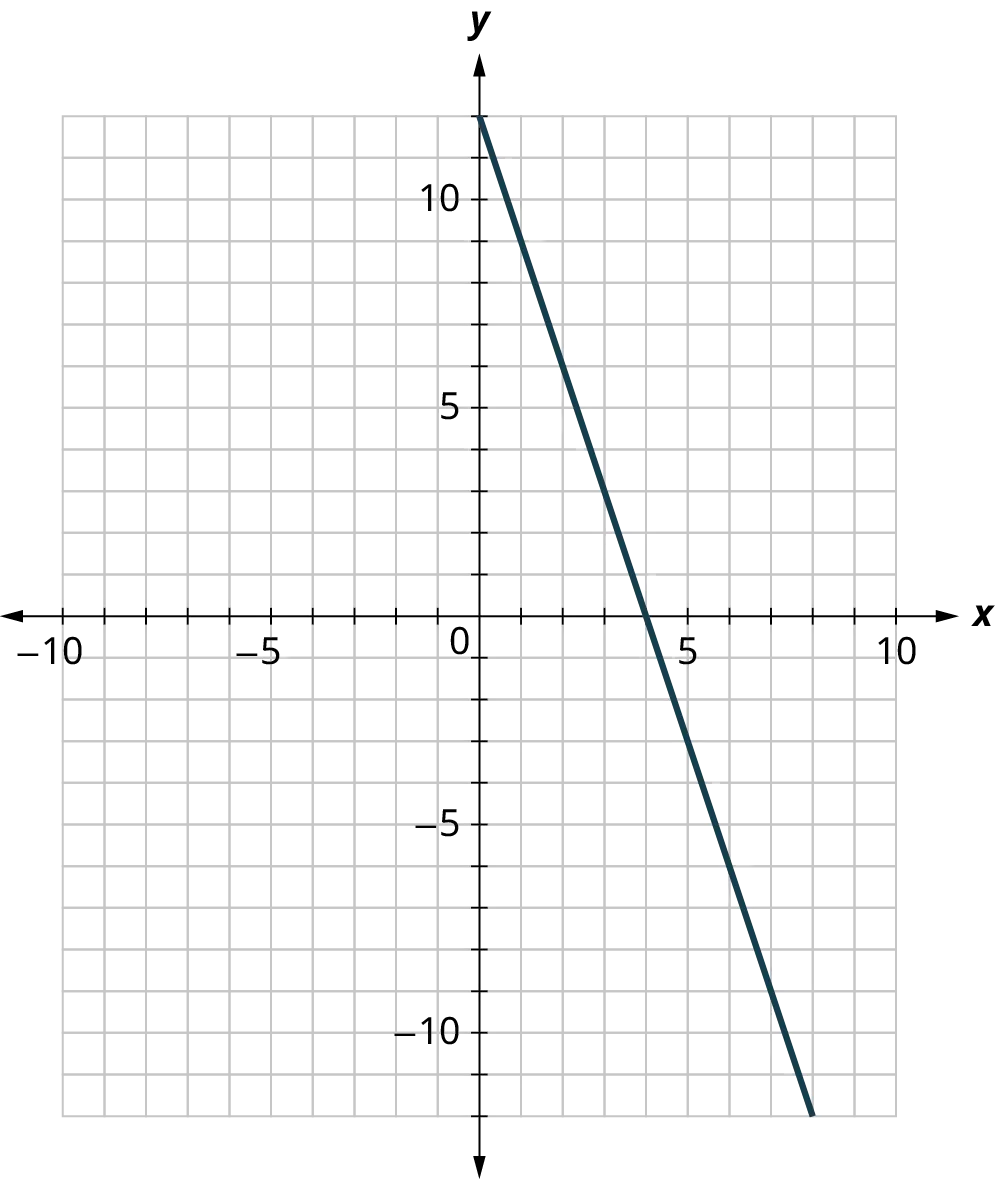5.72 1. $m = \frac{{ - 4}}{3}$ 5.73 1. ${\text{slope}} = - 1$ 5.74 1. slope $m = 2$ and $y{\text{-intercept}} = (0,-1)$ 2. slope $m = - \frac{1}{4}$ and $y{\text{-intercept}} = (0,4)$ 5.75 1.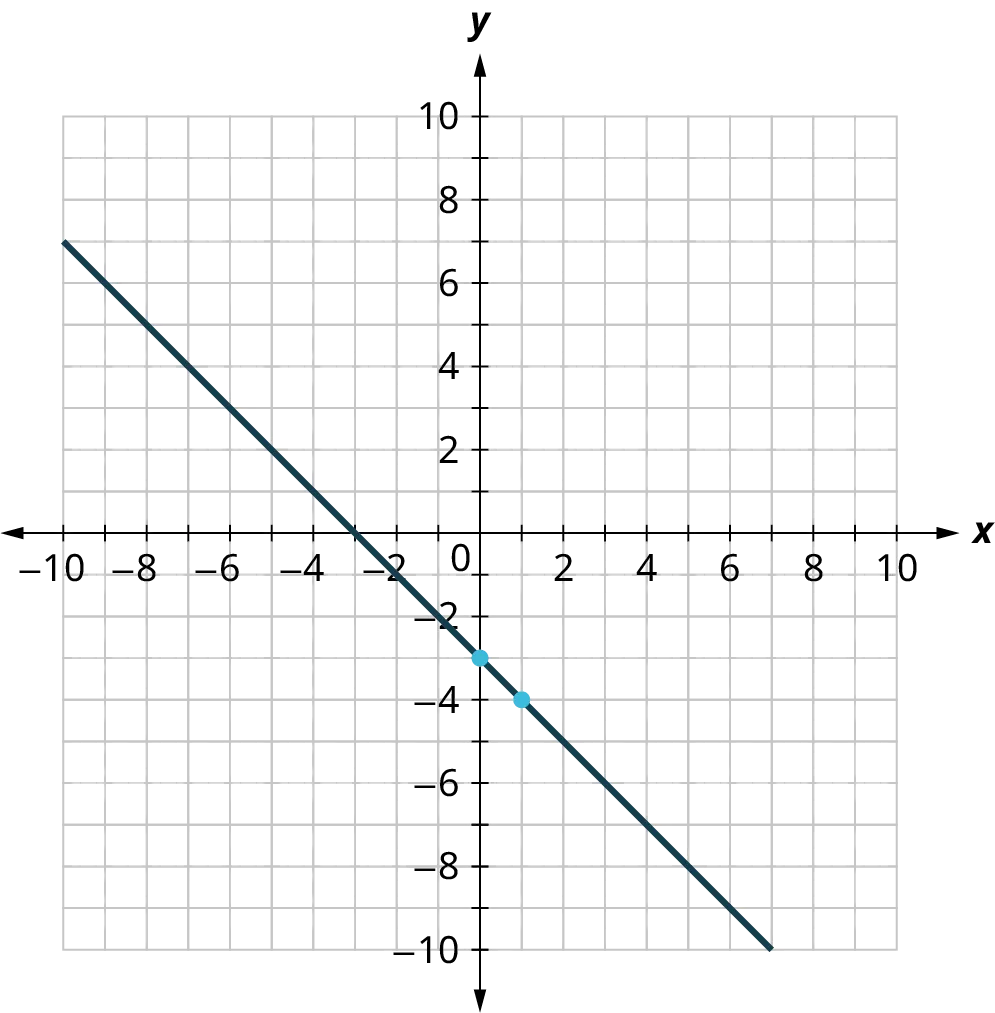5.76 1.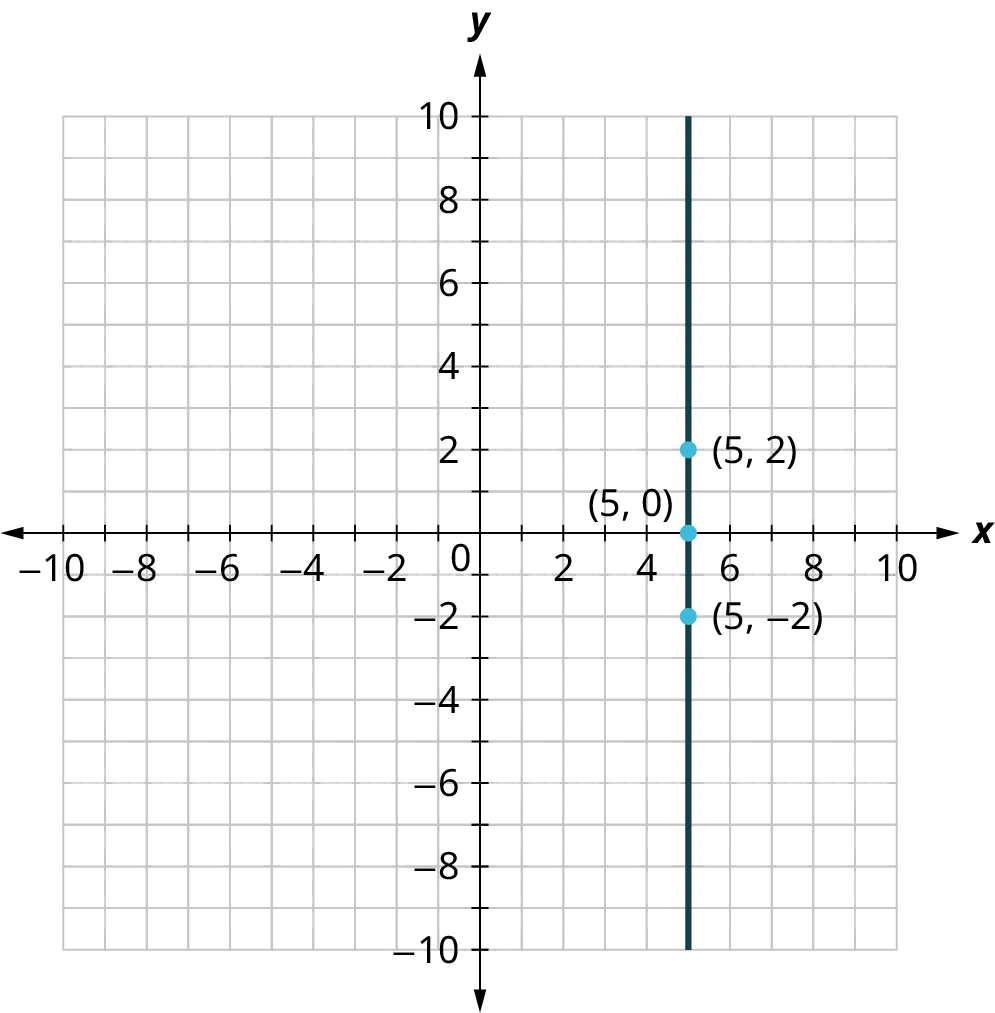5.77 1.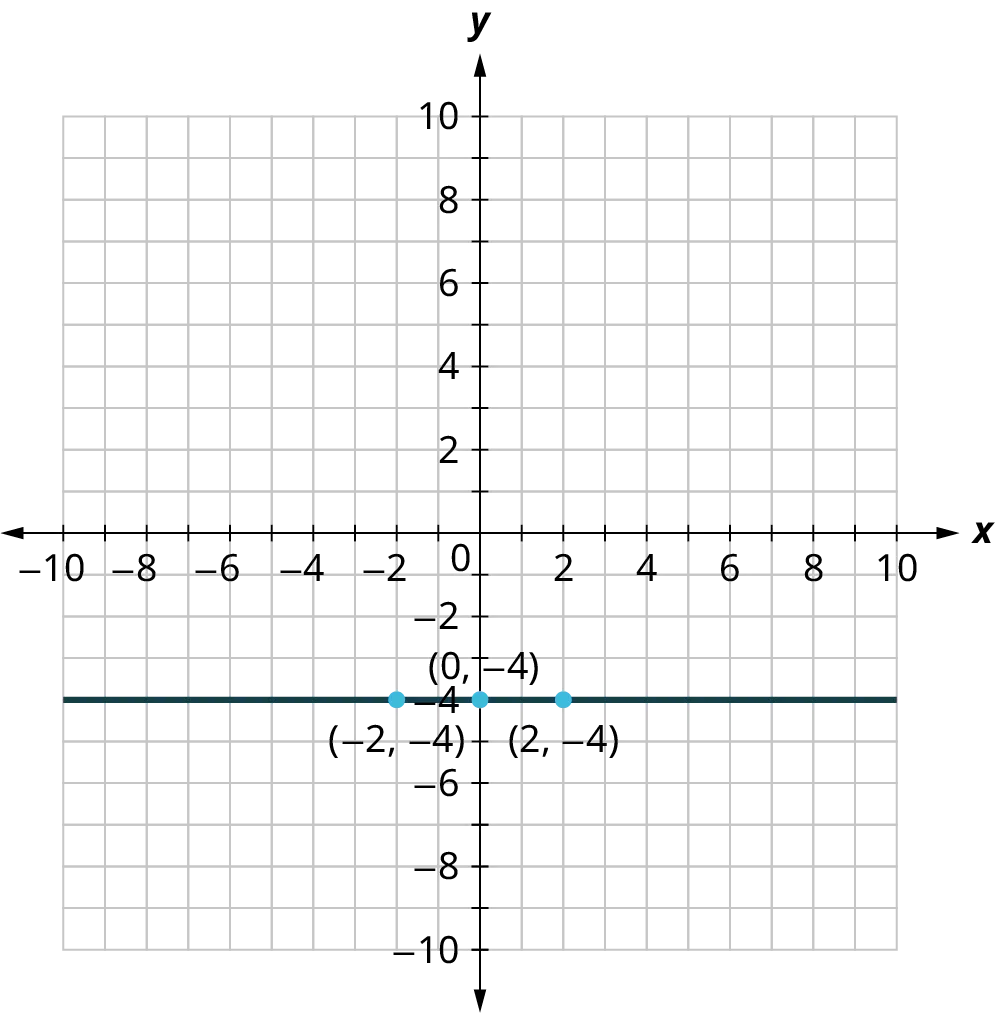5.78 1. (0, 20) is the $y\text{-intercept}$ and represents that there were 20 teachers at Jones High School in 1990. There is no $x\text{-intercept}$. 2. In the first 5 years the slope is 2; this means that on average, the school gained 2 teachers every year between 1990 and 1995. Between 1995 and 2000, the slope is 4; on average the school gained 4 teachers every year. Then the slope is 0 between 2000 and 2005 meaning the number of teachers remained the same. There was a decrease in teachers between 2005 and 2010, represented by a slope of –2. Finally, the slope is 4 between 2010 and 2020, which indicates that on average the school gained 4 teachers every year. 3. Answers will vary. Jones High School was founded in 1990 and hired 2 teachers per year until 1995, when they had an increase in students and they hired 4 teachers per year for the next 5 years. Then there was a hiring freeze, and no teachers were hired between 2000 and 2005. After the hiring freeze, the student population decreased, and they lost 2 teachers per year until 2010. Another surge in student population meant Jones High School hired 4 new teachers per year until 2020 when they had 80 teachers at the school. 5.79 1. 50 inches 2. 66 inches 3. The slope, 2, means that the height $h$ increases 2 inches when the shoe size(s) increases 1 size. 4. The $h$-intercept means that when the shoe size is 0, the woman’s height is 50 inches.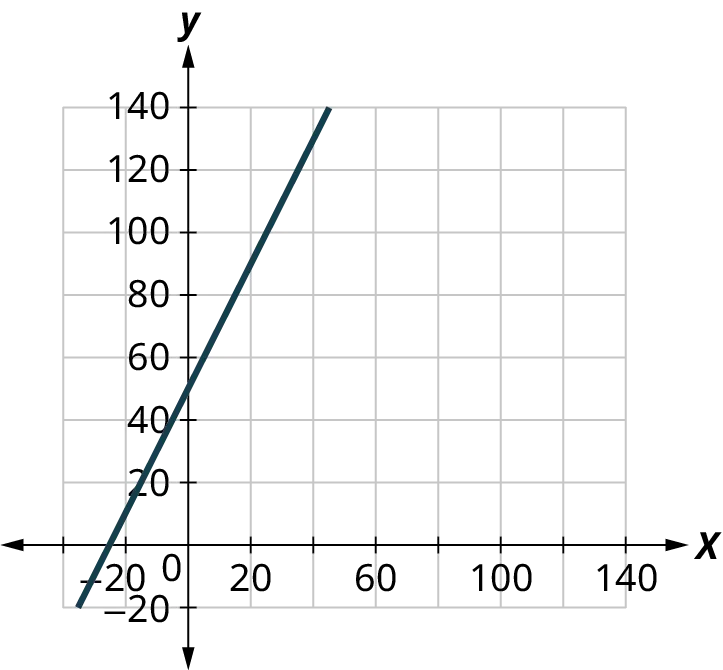5.80 1.$25
2.
$85 3. The slope, 4, means that the weekly cost, $C$, increases by$4 when the number of pizzas sold, $p$, increases by 1. The $C$-intercept means that when the number of pizzas sold is 0, the weekly cost is $25. 4. Graph: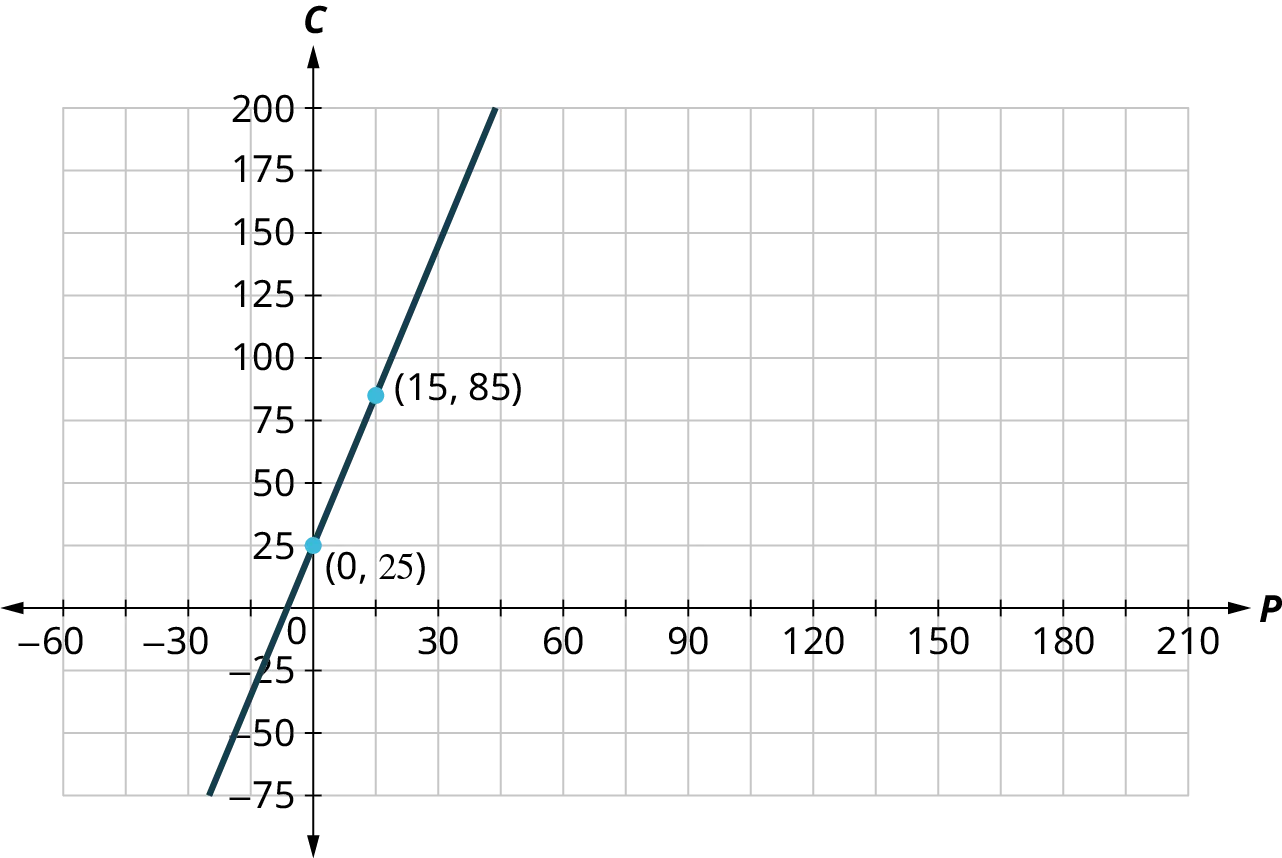5.81 1. No 2. Yes 5.82 1. No 2. No 5.83 1.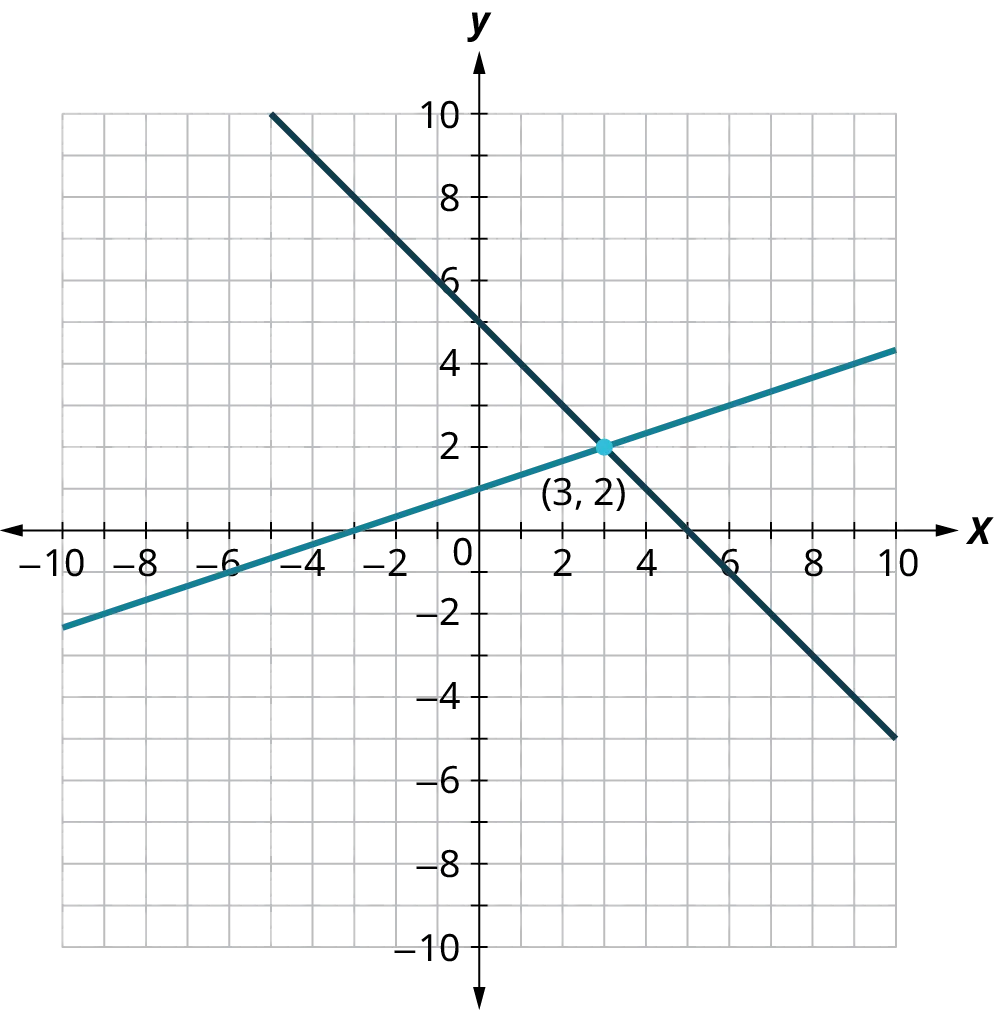5.84 1. (3, 2) 5.85 1. $(3, 2)$ 5.86 1. There is no solution to this system. 5.87 1. There are infinitely many solutions to this system. 5.88 1. Jenna burns 8.3 calories per minute circuit training and 11.2 calories per minute while on the elliptical trainer. 5.89 1. not a solution 2. a solution 5.90 1. The region containing (0, 0) is the solution to the system of linear inequalities. 5.91 1. The solution is the darkest shaded region.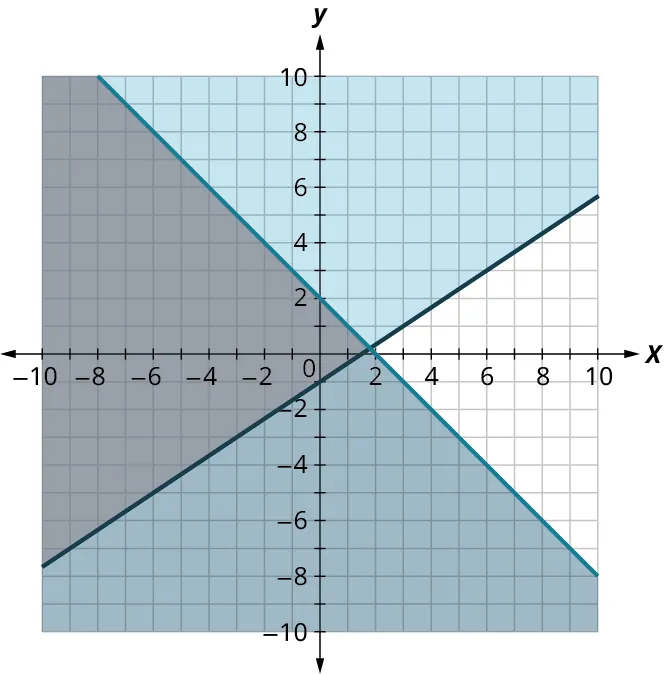5.92 1. The solution is the lighter shaded region.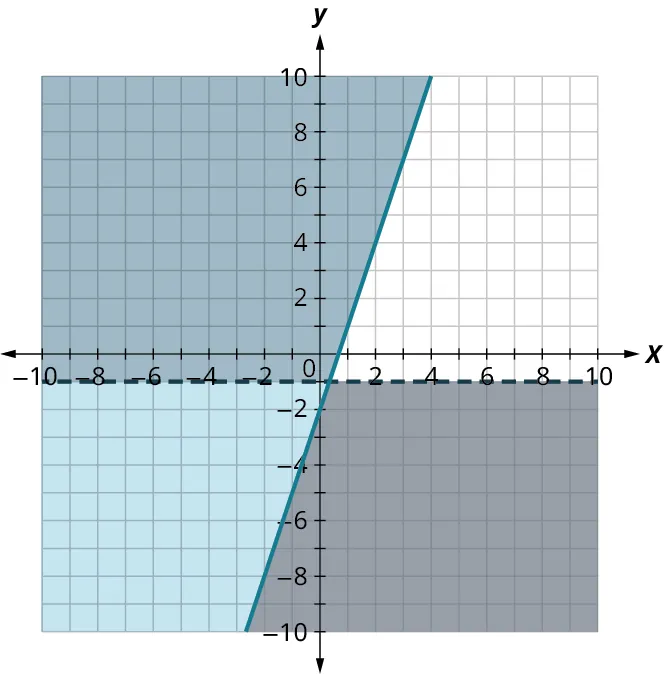5.93 1. No solution.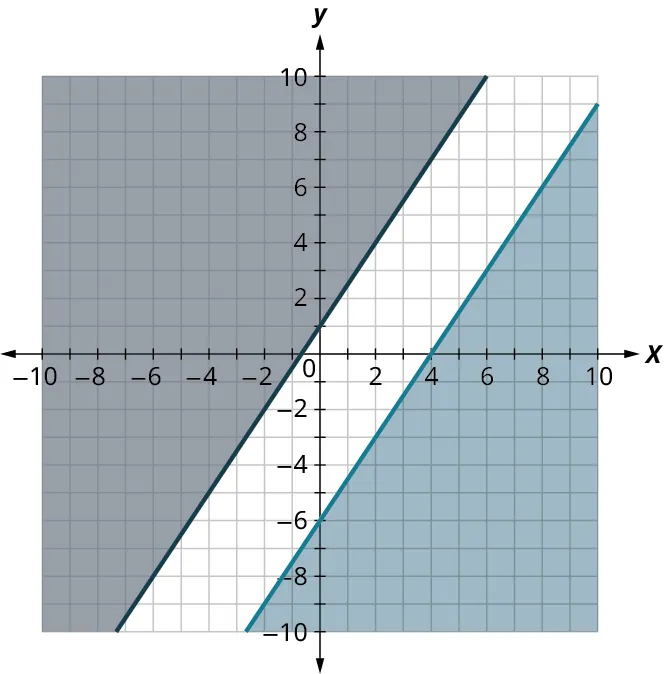5.94 1. The solution is the lighter shaded region.5.95 1. $\left\{ \begin{array}{l}240h + 160C \ge 800\\1.40h + 0.50C \le 5\\h \ge 0\\C \ge 0\end{array} \right.$ 2.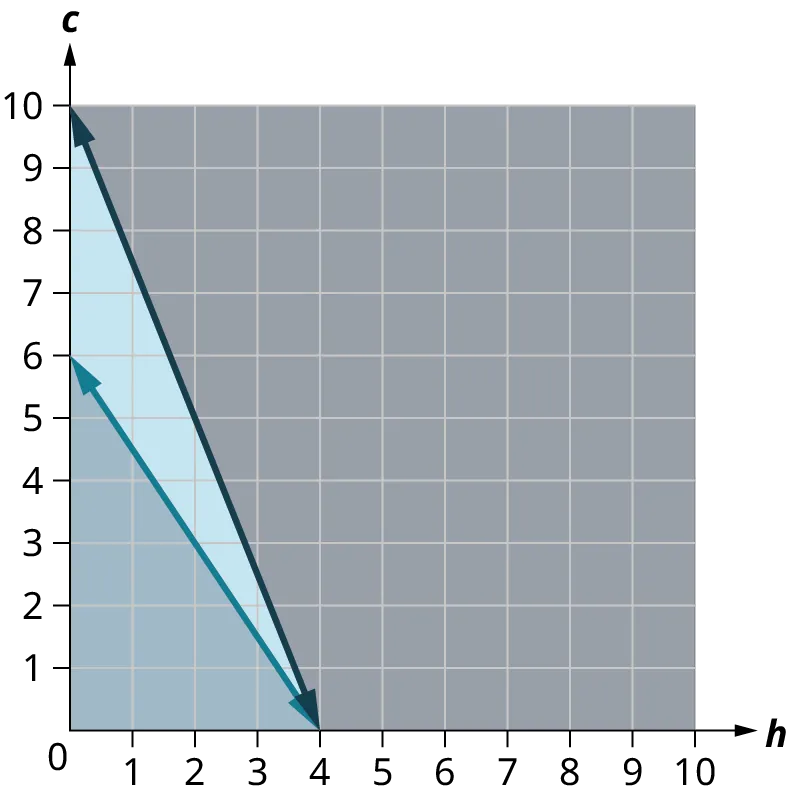3. The point $(3, 2)$ is not in the solution region. Omar would not choose to eat 3 hamburgers and 2 cookies. 4. The point $(2, 4)$ is in the solution region. Omar might choose to eat 2 hamburgers and 4 cookies. 5.96 1. With $a =$ the number of bags of apples sold, and $b =$ the number of bunches of bananas sold, the objective function is $P = 4a + 6b$. 5.97 1. $T = 20x + 28y$ 5.98 1. The constraints are $a + b \leq 20$ and $3a + 5b \leq 70$. The summary is: $P = 4a + 6b$, $a + b \leq 20$, and $3a + 5b \leq 70$. 5.99 1. The constraints are: $15 \leq x \leq 22$ $13 \leq y \leq 19$ So the system is: $T = 20x + 28y$ $15 \leq x \leq 22$ $13 \leq y \leq 19$ 5.100 1. The maximum value for the profit $P$ occurs when $x = 15$ and $y = 5$. This means that to maximize their profit, the Robotics Club should sell 15 bags of apples and 5 bunches of bananas every day. ### Check Your Understanding 1. $J = V + 2$, $V = J - 2$ 2. $5x+8$, $2n+3m$ 3. $(8x + 12x) \div \left( {4x - 2x} \right)$ 4. $- 3$ 5. ${x^2}-2xy + {y^2}$ 6. $3x\left( {3{x^2} + x-2} \right)$ 7. multiplication 8. division 9. It is a correct solution strategy. Let $\begin{array}{rcl}{x}&{ = }&{38}\\{8\left( {38-2} \right)}&{ \mathop = \limits^? }&{6\left( {38 + 10} \right)}\\{8\left( {36} \right)}&{ \mathop = \limits^? }&{6\left( {48} \right)}\\{288}&{ = }&{288 ✓}\\\end{array}$ 10. It is a correct solution strategy. Let $\begin{array}{rcl}{x}&{ = }&{- \,2}\\{7 + 4\left( {2 + 5\left( { - \,2} \right)} \right)}&{ \mathop = \limits^? }&{3\left( {6\left( { -\,2} \right) + 7} \right)-\left( {13\left( { -\,2} \right) + 36} \right)}\\{7 + 4\left( {2-10} \right)}&{ \mathop = \limits^? }&{3\left( { - 12 + 7} \right)-\left( { -\,26 + 36} \right) }\\{7 + 4\left( { -\,8} \right)}&{ \mathop = \limits^? }&{3\left( { - 5} \right)-\left( {10} \right) }\\{7-32}&{ \mathop = \limits^? }&{ -\,15-10}\\{-\,25}&{ = }&{-\,25✓}\end{array}$ 11. This is not a correct solution strategy. The negative sign is not distributed correctly in the second line of the solution strategy. The second line should read $8x + 7-2x + 9 = 22-4x + 4$. 12. ${{T}} = 1.7x + 3$ 13.$40.40
14.
${{T}} = 1.6y + 5$
15.
$40.20 16. The Enjoyable Cab Company, because the cab fare will be$ 0.20 less than what it would cost to take a taxi from the Nice Cab Company.
17.
Luis is; there are infinitely many solutions. If this is solved using the general strategy, it simplifies to $0 = 0$. This is a true statement, so there are infinitely many solutions.
18.
d $F = \frac{9}{5}C + 32$
19.
b $K = C + 273$, although d $C = K – 273$ is an equivalent formula.
20.
a $K = \frac{5}{9}\left( {F-32} \right) + 273$
21.
b $R = \frac{9}{5}C + 492$
22.
d $\left( { - \infty , - 1} \right)$
23.
b $[ - 5,\infty )$
24.
c $\left[ {\frac{3}{2},\infty } \right)$
25.
a $( - 4,3)$
26.
e $6x \geq 24$
27.
b $-6x \gt 18$
28.
c $4x - 3 > - 11$
29.
c $- 3x + 14 > - 13$
30.
b $8x < 764$
31.
d $50x > \text{8,120}$
32.
a True
33.
a True
34.
a True
35.
b False
36.
a True
37.
$12{:}16$
38.
$16{:}28$
39.
324.00 British pounds (None of these.)
40.
10 inches
41.
Yes he can, but barely. At 37 miles per gallon, Albert can drive $499.5$ miles. While in theory he can make it, he probably should fill up with gasoline somewhere along the way!
42.
$40.66$
43.
d $y = \frac{5}{3}$
44.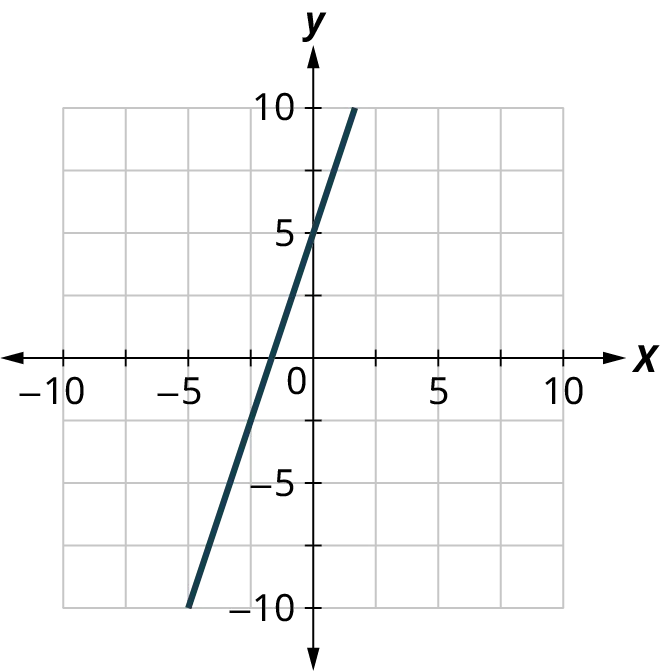45.
b $y = \frac{1}{2}x + 4$
46.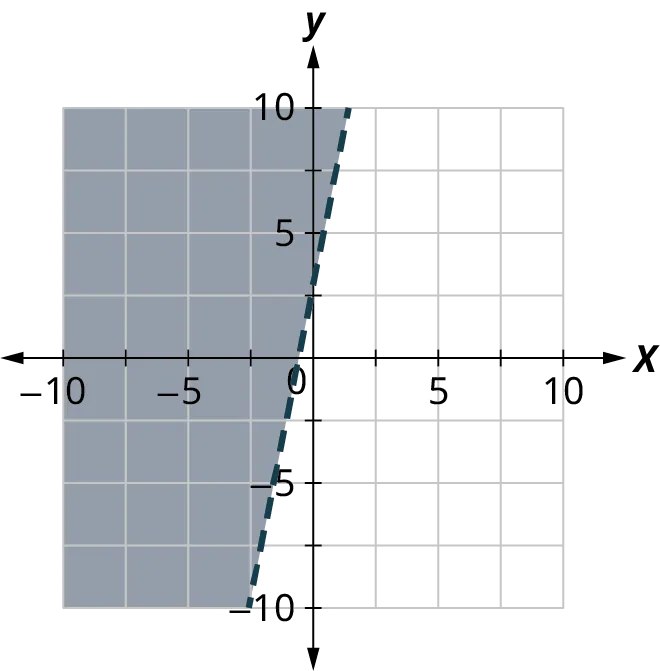47.
b $y \leq -2x + 5$
48.
d
49.
b
50.
c
51.
d
52.
b False
53.
b False
54.
a True
55.
b False
56.
$x = \frac{5}{2} \pm \frac{{\sqrt 5 }}{2}$
57.
a True
58.
b False
59.
b False
60.
a True
61.
b False
62.
a True
63.
b False
64.
b False
65.
a True
66.
b False
67.
Elimination
68.
Substitution
69.
Substitution
70.
Elimination
71.
Substitution
72.
Elimination
73.
Elimination
74.
Substitution
75.
c
76.
e
77.
d
78.
b
79.
a
80.
c
81.
a
82.
b
83.
d
84.
a
85.
c
86.
d
87.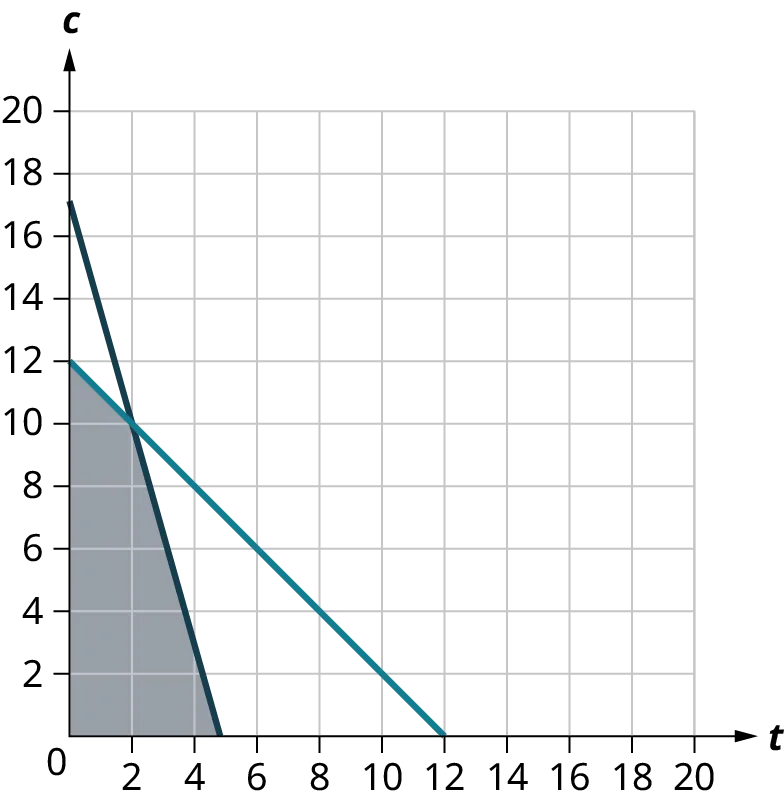88.
b
89.
$140$
Order a print copy

As an Amazon Associate we earn from qualifying purchases.